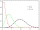# Logarithm + area - math problems

#### Number of problems found: 1

• Poisson distribution - daisiesThe meadow behind FLD was divided into 100 equally large parts. Subsequently, it was found that there were no daisies in ten of these parts. Estimate the total number of daisies in the meadow. Assume that daisies are randomly distributed in the meadow.

We apologize, but in this category are not a lot of examples.
Do you have an exciting math question or word problem that you can't solve? Ask a question or post a math problem, and we can try to solve it.

We will send a solution to your e-mail address. Solved examples are also published here. Please enter the e-mail correctly and check whether you don't have a full mailbox.

Logarithm - math problems. Area - math problems.